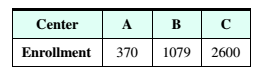# College Security A college apportions 40 security personnel among three education centers according to their enrollments. The following table shows the present enrolments at the three centers. a. Use the Hamilton method to apportion the security personnel. b. After one semester, the centers have the following enrolments, Center A has an increased enrollment of 14 students, which is an increase of 14 356 ≈ 0.039 = 3.9 % . Center B has an increased enrollment of 25 students, which is an increase of 25 1054 ≈ 0.024 = 2.4 % . If the security personnel are reapportioned using the Hamilton method, will the population paradox occur? Explain. Center A has an increased enrollment of 14 students,### Mathematical Excursions (MindTap C...

4th Edition
Richard N. Aufmann + 3 others
Publisher: Cengage Learning
ISBN: 9781305965584

#### Solutions

Chapter
Section### Mathematical Excursions (MindTap C...

4th Edition
Richard N. Aufmann + 3 others
Publisher: Cengage Learning
ISBN: 9781305965584
Chapter 4.1, Problem 18ES
Textbook Problem
1 views

## College Security A college apportions 40 security personnel among three education centers according to their enrollments. The following table shows the present enrolments at the three centers.a. Use the Hamilton method to apportion the security personnel.b. After one semester, the centers have the following enrolments,Center A has an increased enrollment of 14 students, which is an increase of 14 356 ≈ 0.039 = 3.9 % . Center B has an increased enrollment of 25 students, which is an increase of 25 1054 ≈ 0.024 = 2.4 % . If the security personnel are reapportioned using the Hamilton method, will the population paradox occur? Explain. Center A has an increased enrollment of 14 students,

To determine

(a)

Use the Hamilton method to apportion the security personnel.

### Explanation of Solution

Given Information:

 Center A B C Enrollment 356 1054 2590

Concept used:

Standard Divisor=Total EnrollmentNumber of security personnel to apportion

Calculation:

Since a college apportions 40 security personnel first we calculate standard divisor Standard Divisor=Total EnrollmentNumber of security personnel to apportion

Therefore,

Standard Divisor=356+1054+259040=400040=100

Now in Hamilton method divide each enrollment by standard divisor to get a quotient and standard quota

 Center Enrollments Quotient Standard quota Number of Security personnel A 356 356100=3
To determine

(b)

If the security personnel are reapportioned using the Hamilton method, will the population paradox occur? Explain.

### Still sussing out bartleby?

Check out a sample textbook solution.

See a sample solution

#### The Solution to Your Study Problems

Bartleby provides explanations to thousands of textbook problems written by our experts, many with advanced degrees!

Get Started

Find more solutions based on key concepts
In Exercises 1-8, use the graph of the given function f to determine limxaf(x) at the indicated value of a, if ...

Applied Calculus for the Managerial, Life, and Social Sciences: A Brief Approach

2. Define the terms population, sample, parameter and statistic.

Statistics for The Behavioral Sciences (MindTap Course List)

Divide the following fractions and reduce to lowest terms. 23.

Contemporary Mathematics for Business & Consumers

Construct a 33 matrix A having the property that A=AT. What special characteristic does A have?

Finite Mathematics for the Managerial, Life, and Social Sciences

Which curve is simple but not closed?

Study Guide for Stewart's Multivariable Calculus, 8th

Use a Table of Integrals to evaluate .

Study Guide for Stewart's Single Variable Calculus: Early Transcendentals, 8th

If D is defined as in (9), then find eDt.

A First Course in Differential Equations with Modeling Applications (MindTap Course List)

Test the following hypotheses by using the χ2 goodness of fit test. H0: pA = .40, pB = .40, and pC = .20 Ha: Th...

Modern Business Statistics with Microsoft Office Excel (with XLSTAT Education Edition Printed Access Card) (MindTap Course List)

Reminder Round all answers to two decimal places unless otherwise indicated. Marginal Tax RateLet T(I) denote t...

Functions and Change: A Modeling Approach to College Algebra (MindTap Course List)